# Math Operators Object Detection (Video Demo)

### Test 1 - Simple Math Problems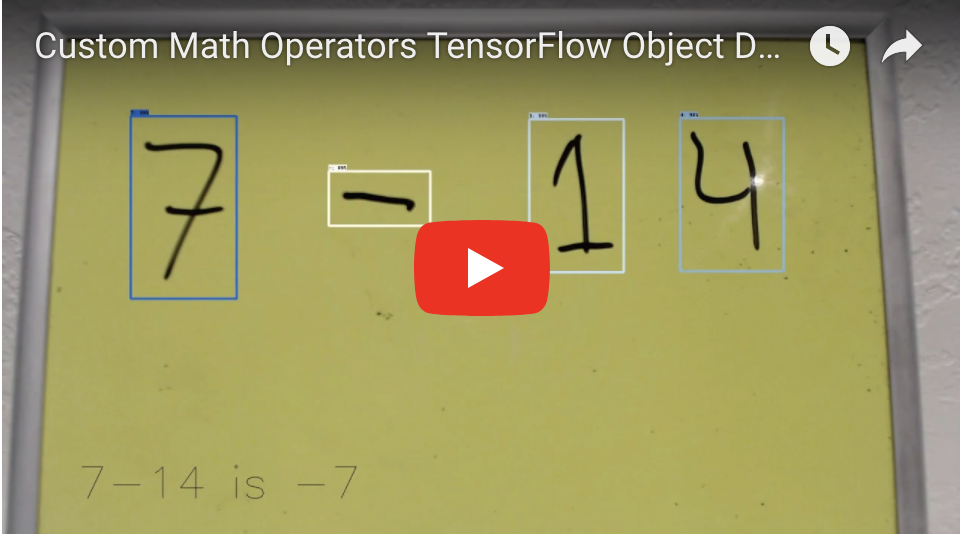### Test 2 - Linear AlgebraUtilizing TensorFlow Object Detection API open source framework makes it feasible to construct, train and deploy a custom object detection model with ease. The detection model shown above uses TensorFlow's API and detects handwritten digits and simple math operators. In addition, the output of the predicted objects (numbers & math operators) are then evaluated and solved. Currently, the model created above is limited to basic math and linear algebra.

# Model

Model: ssd_mobilenet_v1_coco_2017_11_17
Config: ssd_mobilenet_v1_coco

### SSD Mobilenet Architecture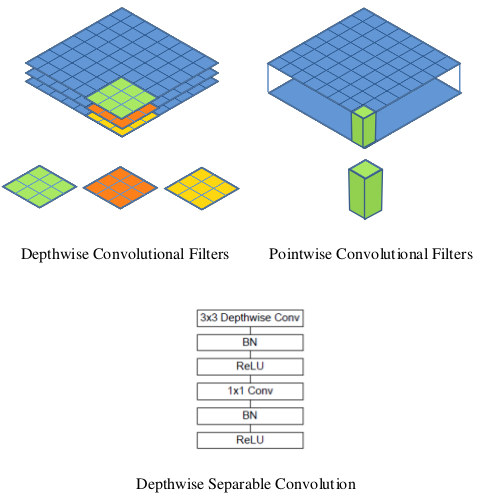• Convolution Neural network - The convolutional layer is the core building block of a CNN. The layer's parameters consist of a set of learnable filters (or kernels), which have a small receptive field, but extend through the full depth of the input volume. During the forward pass, each filter is convolved across the width and height of the input volume, computing the dot product between the entries of the filter and the input and producing a 2-dimensional activation map of that filter. As a result, the network learns filters that activate when it detects some specific type of feature at some spatial position in the input. (Source: Wikipedia)

• Batch Normalization - Batch normalization potentially helps in two ways: faster learning and higher overall accuracy. The improved method also allows you to use a higher learning rate, potentially providing another boost in speed. Normalization (shifting inputs to zero-mean and unit variance) is often used as a pre-processing step to make the data comparable across features. As the data flows through a deep network, the weights and parameters adjust those values, sometimes making the data too big or too small again - "internal covariate shift". By normalizing the data in each mini-batch, this problem is largely avoided. (Source: Derek Chan ~ Quora)

• Rectified linear unit or (ReLU) - ReLu is an activation function. In biologically inspired neural networks, the activation function is usually an abstraction representing the rate of action potential firing in the cell. In its simplest form, this function is binary—that is, either the neuron is firing or not. ReLU is half rectified from the bottom. It is f(s) is zero when z is less than zero and f(z) is equal to z when z is above or equal to zero. With a range of 0 to infinity. (Source: Towards Data Science)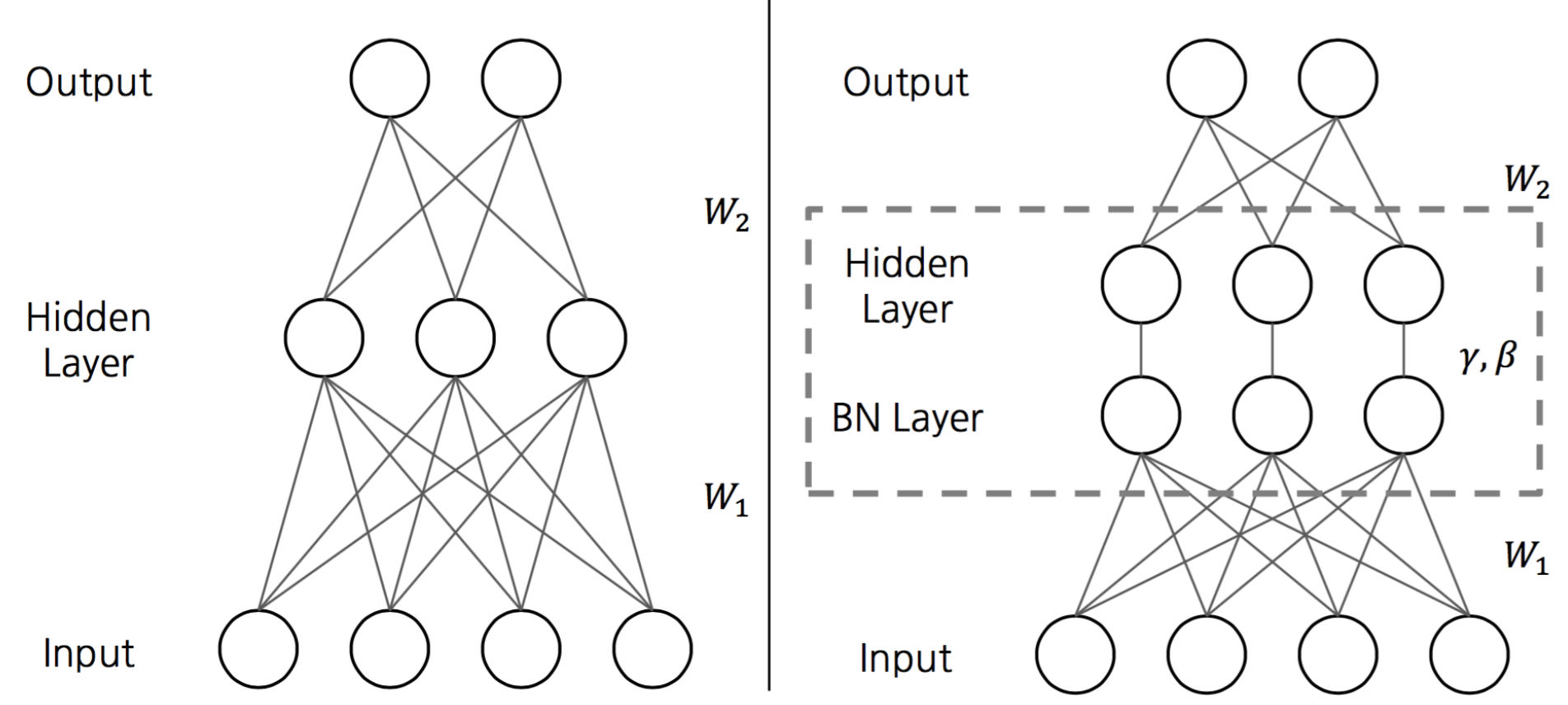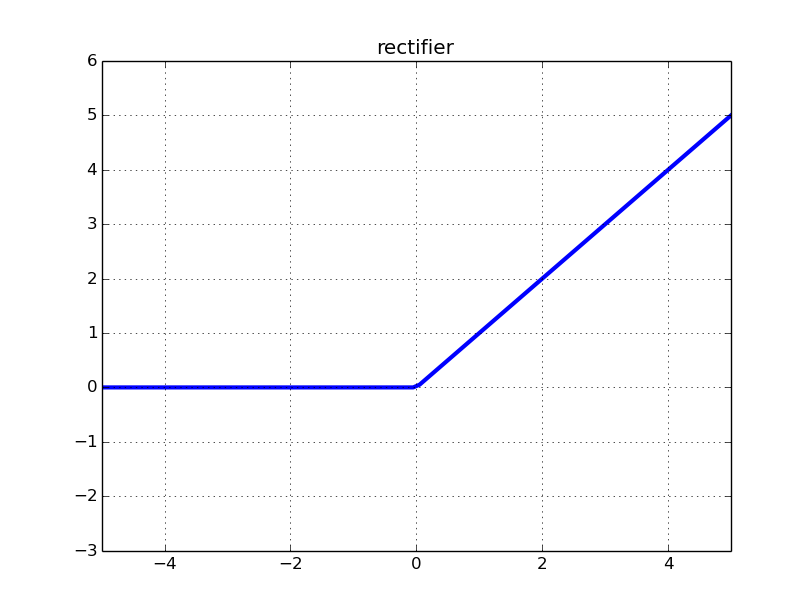# Summary of training this model

#### Step 1:

Create an image library - The pre-existing ssd_mobilenet_v1_coco model was trained with a custom, created from scratch, image library (of math numbers & operators). This image library can be substituted with any object or objects of choice. Due to the constraint of time, the model above was trained on a total of 345 images of which 10% was allocated for test validation.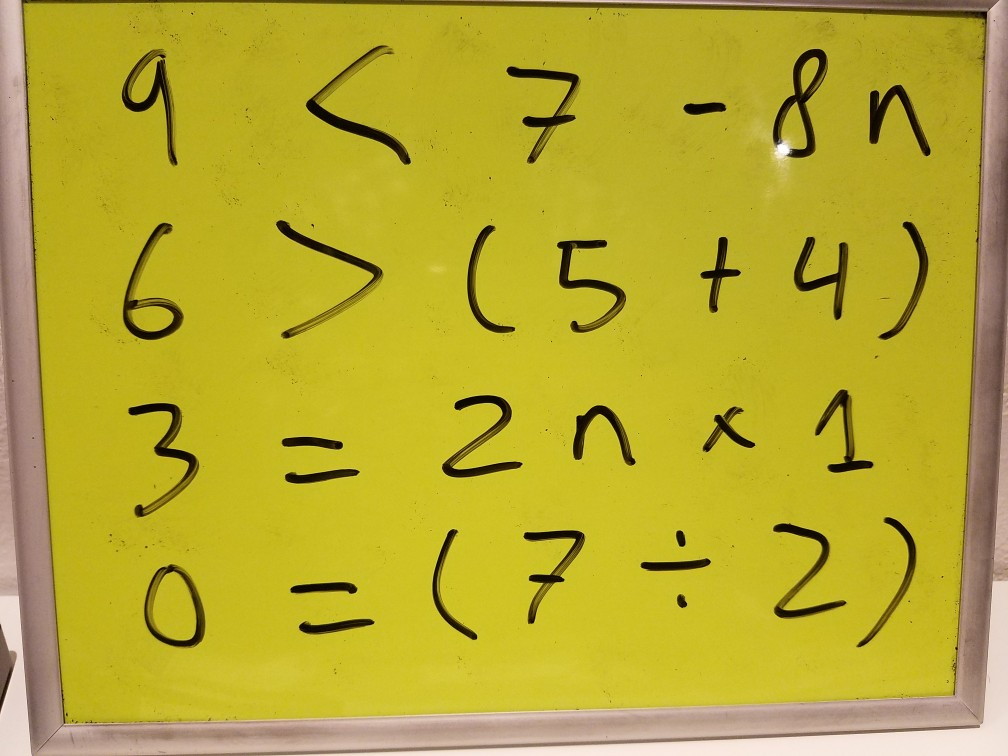#### Step 2:

Box & label each class - In order to train and test the model, TensorFlow requires that a box is drawn for each class. To be more specific, it needs the X and Y axis (ymin, xmin, ymax, xmax) of the box in relation to the image. These coordinates is then respectively divided by the lenght or width of the image and is stored as a float. An example of the process is shown below. (Note: the current model contains 23 classes) Thanks to tzutalin tzutalin, labelImg, with the creation of GUI that makes this process easy.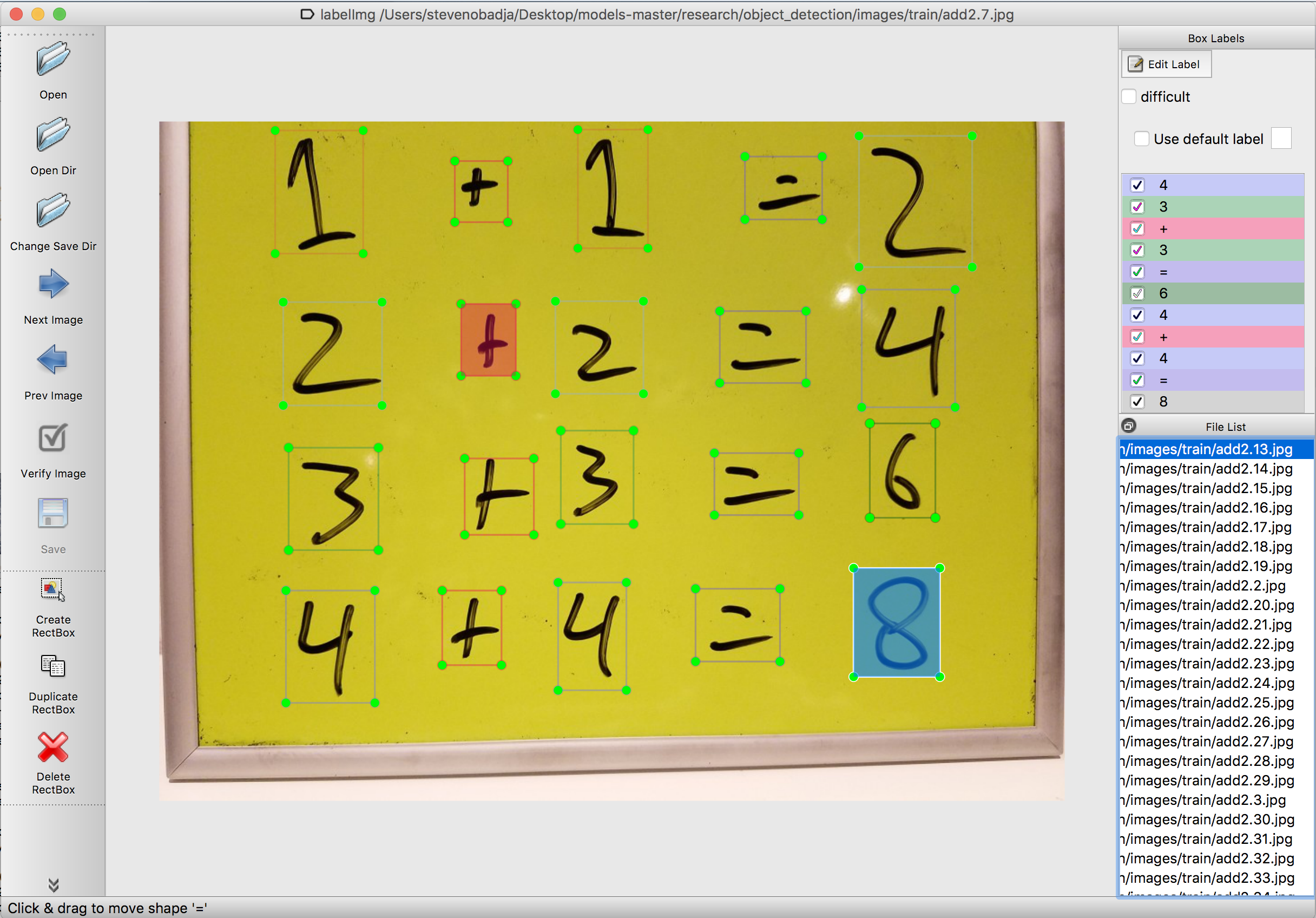#### Step 3:

Convert files - Once the labeling process is complete the folder will be full with XML files, however this cannot be used yet by TensorFlow for training and testing. Instead the XML files needs to be converted into a CSV file. Then the CSV file will then be converted to tfrecords file for training.

#### Step 4:

Create pbtxt - Create a pbtxt file by creating ID's and Name (labels) for each class. This file will be used with the finished model as an category_index.

#### Step 5:

Train the model - (See model above)
Summary: input layer --> 3x3 CNN --> batch normalization --> activation function: ReLu --> 1x1 CNN --> batch normalization --> activation function: ReLu --> output layer.

After the output layer, it compares the output to the intended output --> cost function (weighted_sigmoid) --> optimization function (optimizer) --> minimize cost (rms_prop_optimizer, learning rate = 0.004)

1 cycle of summary above = 1 Global Step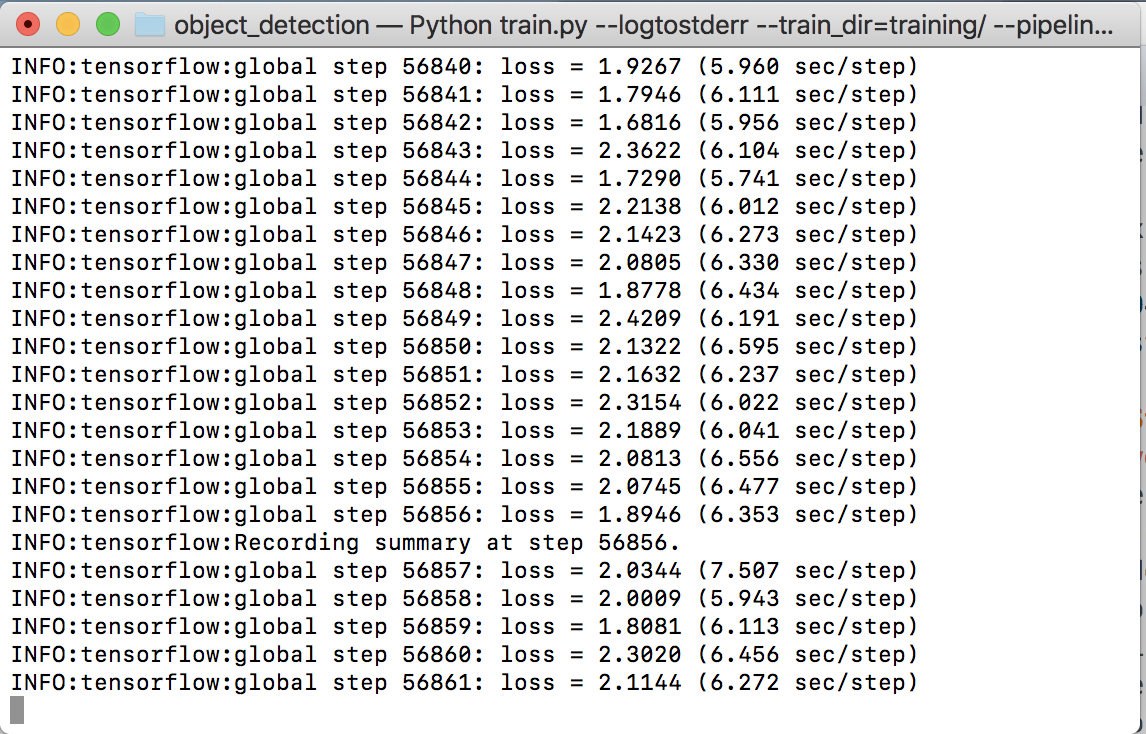This process requires heavy computing power, due to the constraints of hardware (CPU only), it took approximately 4 days & 7 hours to complete 50k Global Step.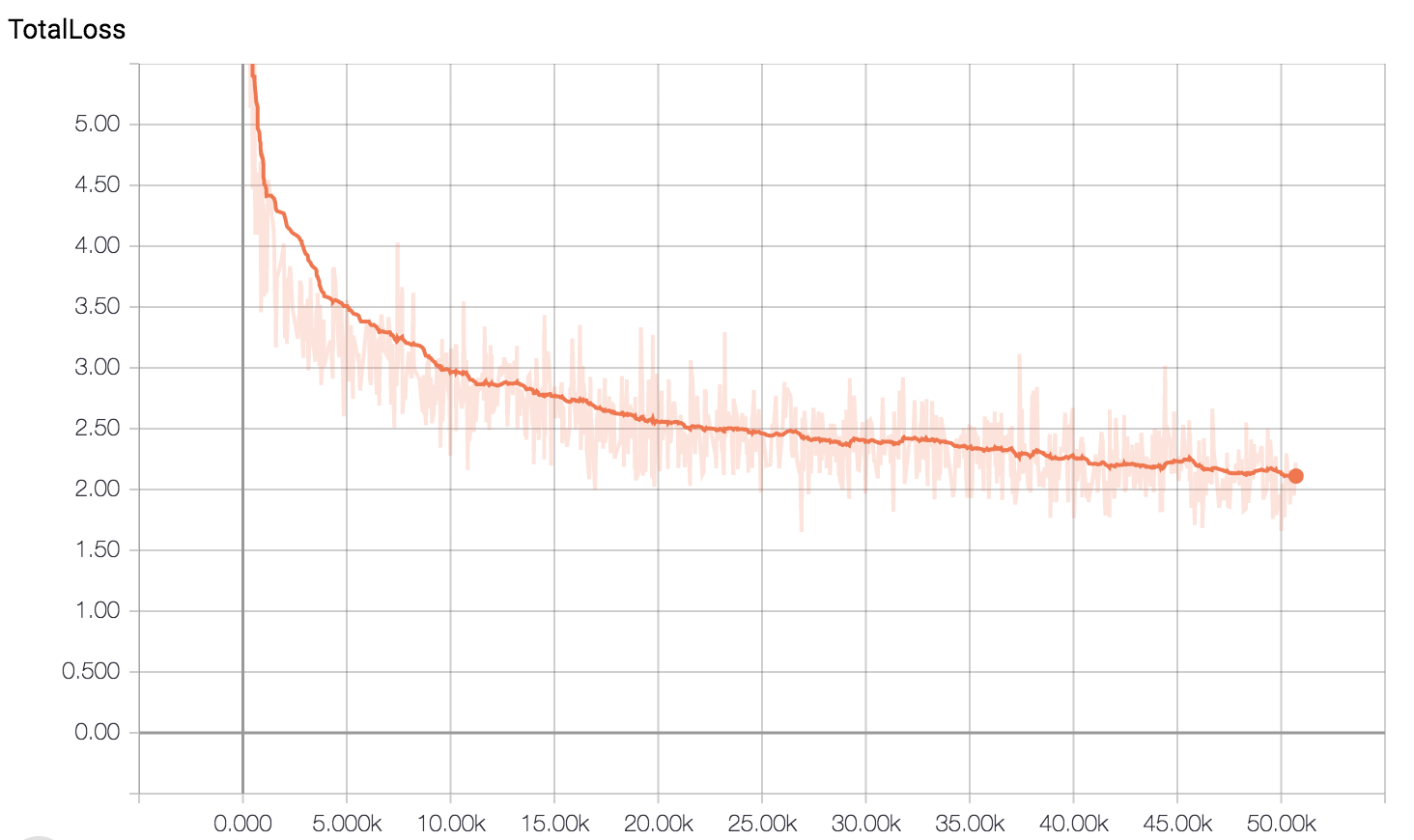#### Step 6:

Export inference graph - Once a model is trained with an acceptable loss rate. It is stopped by the user manually. As the model is being trained it is creates a checkpoint file after each set milestone. This checkpoint file is then converted into an inference graph which is used for deployment/serving.

# Source & Support Files

Will contain:

• train.py
• export_inference_graph.py
• All other support docs...

Will contain:

• ssd_mobilenet_v1_coco_2017_11_17 (model used for this demo)
• All other models released by Tensorflow...

Will contain:

• ssd_mobilenet_v1_coco (config used for this demo)
• All other configs released by Tensorflow...

Will contain:

• xml_to_csv.py
• generate_tfrecord.py

Will contain:

• labelImg.py

# Special Thanks!

To Harrison Kinsley (Sentdex)

Get A Weekly Email With Trending Projects For These Topics
No Spam. Unsubscribe easily at any time.
Python (1,126,520
Python3 (32,500
Tensorflow (12,575
Object Detection (2,486
Detection (1,182
Linear Algebra (812
Tensorflow Tutorials (440
Tensorflow Examples (290
Tensorflow Models (276
Tensorflow Experiments (171
Coco (145
Related Projects